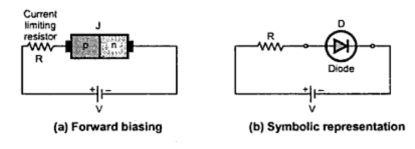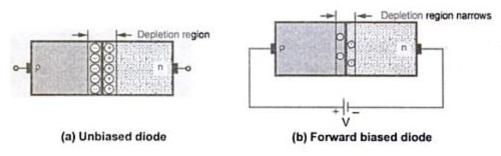### Forward Biasing of P-N junction Diode

If am external d.c. voltage is connected in such a way that the p-region terminal is connected to the positive of the d.c. voltage and the n-region is connected to the negative of the d.c. voltage, the biasing condition is called forward biasing. The p-n junction is said to be forward biased.
Note : Forward biasing means connecting p-region to +ve and n-region to -ve of the battery.Fig. 1
The Fig. 1(a) shows the connection of forward biasing of a p-n junction. To limit the current, practically a current limiting resistor is connected in series with the p-n junction diode. The Fig. 1(b) shows the symbolic representation of a froward biased diode.
1.1 Operation of Forward Biased Diode
When the p-n junction is forward biased as long as the applied voltage is less than the barrier potential, there can not be any conduction.
When the applied voltage becomes more than the barrier potential, the negative terminal of battery pushes the free electrons against barrier potential from n to p-region. Similarly positive terminal pushes the holes from p to n-region. Thus holes get repelled by positive terminal and cross the junction against barrier potential. Thus the applied voltage overcomes the barrier potential. This reduces the width of depletion region.
As forward voltage is increased, at a particular value the depletion region becomes very much narrow such that large number of majority charge carriers can cross the junction.
The large number of majority carriers constitute a current called forward current. Once the conduction electrons enter the p-region, they become valence electrons. Then they move from hole to hole towards the positive terminal of the battery. The movement of valence electrons is nothing but movement of holes in opposite direction to that of electrons, in the p-region. So current in the p-region is the movement of holes which are majority carriers. This is the hole current. While the current inn the n-region is the movement of free electrons which are majority carriers. This is the electron current. Hence the overall forward current is due to the majority charge carriers. The action is shown in the Fig. 2. These majority carriers can then travel around the closed circuit and a relatively large current flows. The direction of flow of electrons is from negative to positive of the battery. While direction of the conventional current is from positive to negative of the battery as shown in the Fig. 2.Fig. 2   Forward current in a diode
Note : The direction of low of electrons and conventional current is opposite to each other.
1.2 Effect on the Depletion Region
Due to the forward bias voltage, more electrons flow into the depletion region, which reduces the number of positive ions. Similarly flow of holes reduces the number of negative ions. This reduces the width of the depletion region. This is shown in the Fig. 3.Fig. 3

1.3 Effect of the Barrier Potential
Under the influence of applied forward bias voltage, the free electrons get the energy equivalent to the barrier potential so that they can easily overcome the barrier, which is a sort of a hill and cross the junction. While crossing the junction, the electrons give up the amount of energy equivalent to the barrier potential. This loss of energy produces a voltage drop across the p-n junction which is almost equal to the barrier potential.
Note : The polarities of voltage drop across the p-n junction in forward biased condition are opposite to that of barrier potential but the value is almost equal to the barrier potential.
Due to the internal resistance, there is additional small voltage drop across the diode.
Thus the total voltage drop across a p-n junction diode in a forward biased condition is and it is made up of
1. Drop due to barrier potential
2. Drop due to internal resistance.
V=  Vγ   +  Irf
Note : The total V is of the order of 0.7 V for silicon and 0.3 V for the germanium.
1.4 Forward V-I Characteristics of Diode
The response of p-n junction can be easily indicated with the help of characteristics called V-I characteristics of p-n junction. It is the graph of voltage applied across the p-n junction and the current flowing through the p-n junction.
The Fig. 3 shows the forward biased diode. The applied voltage is V while the voltage across the diode is Vf. The current flowing in the circuit is the forward current If. The graph of forward current against the forward voltage V across the diode is called forward characteristics of a diode.
The forward characteristics of a diode is shown in the Fig. 4.Fig. 4   Forward characteristics of a diode

Basically forward characteristics can be divided into two regions :
1. Region O to P : As long as is less than cut-in voltage (Vγ), the current flowing is very small. Practically this current is assumed to be zero.
2. Region P to Q and onwards : As Vf increases towards Vγ the width of depletion region goes on reducing. When Vf exceeds Vγ i.e. cut-in voltage, the depletion region becomes very thin and current If increases suddenly. This increase in the current exponential as shown in the Fig. 4 by the region P to Q.
The point P, after which the forward current starts increasing exponentially is called knee of the curve.
Note : The normal forward biased operation of the diode is above the knee point of the curve. i.e. in the region P-Q.
The forward current is the conventional current, hence it is treated as positive and the forward voltage  Vf is also treated positive. Hence the forward characteristics is plotted in the first quadrant.
1.5 Forward Resistance of Diode
The resistance offered by the p-n junction diode in forward biased condition is called forward resistance. The forward resistance is defined in two ways :
1) Static forward resistance :
This is the forward resistance of p-n junction diode when p-n junction is used in d.c. circuit and the applied forward voltage is d.c. This resistance is denoted as R and is calculated at a particular point on the forward characteristics.
Thus at a point E shown in the forward characteristics, the static resistance R is defined as the ratio of the d.c. voltage applied across the p-n junction to the d.c. current flowing through the p-n junction.
R = Forward d.c. voltage/Forward d.c. current = OA/OC at point E
2) Dynamic forward resistance :
The resistance offered by the p-n junction under a.c. condition is called dynamic resistance denoted as rf.
Note : The dynamic resistance is reciprocal of the slope of the forward characteristics.
Consider the change in applied voltage from point A to B shown in the Fig. 4. This is denoted as ΔVf. The corresponding change in the forward current is from point C to D. It is denoted as ΔIf. Thus the slope of the characteristics is ΔIf/ΔVf. The reciprocal of the slope is dynamic resistance rf.
r = ΔVf/ΔI=1/(ΔIf/ΔVf) = 1/Slope of forward characteristics
Note : Generally the value of r is very small of the order of few ohms, in the operating region i.e. above the knee.